Total marks: --
Total time: --
INSTRUCTIONS
(1) Assume appropriate data and state your reasons
(2) Marks are given to the right of every question
(3) Draw neat diagrams wherever necessary

Solve any one question from Q1 and Q2
1 (a) For the network shown below, find the current through the 10 Ω resistor using Thevenin's theorem.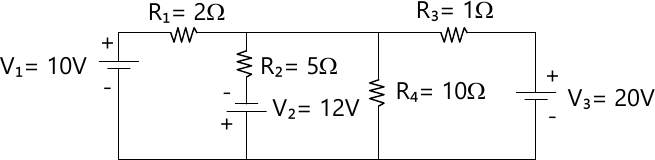6 M
1 (b) A reduced incidence matrix of a graph is given by: $[A]= \begin{bmatrix} 1 &1 &0 &0 &0 &1 \\0 &-1 &1 &-1 &0 &0 \\-1 &0 &-1 &0 &-1 &0 \end{bmatrix}$ Obtain the number of possible trees.
6 M

2 (a) Find the Norton's equivalent circuit across a-b for the network shown: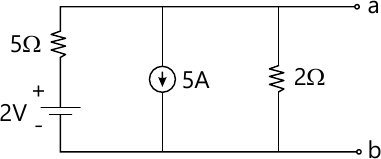6 M
2 (b) Explain the following terms with example:
i) Directed graph
ii) Incidence Matrix
iii) Fundamental Tie-set matrix.
6 M

Solve any one question from Q3 and Q4
3 (a) For the network shown, initially switch is kept open for a long time and closed at t=0. Find expression for VC(t).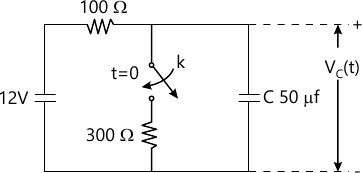6 M
3 (b) Define figure of merit. Prove that the bandwidth of a series resonant circuit is given by: $BW= (f_2 - f_1) = \dfrac {R}{2πL}$
6 M

4 (a) Derive the expression for current i(t) for the series RL circuit shown below.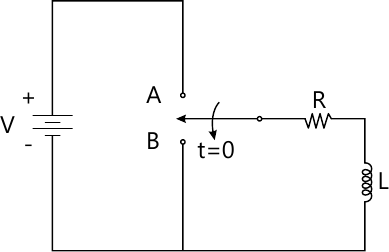6 M
4 (b) For the network shown, determine:
i) Resonance frequency
ii) Quality factor
iii) Half power frequencies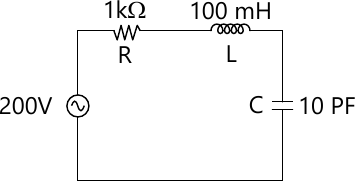6 M

Solve any one question from Q5 and Q6
5 (a) Sketch the reactance curves for a constant K-T section HPF. Also obtain the expression for cut-off frequency.
7 M
5 (b) Derive expression for Z0T in terms of open and short circuit impedances.
6 M

6 (a) What are low pass filter? Derive expression for the cut-off frequency of prototype low pass filter in terms of L and C.
7 M
6 (b) Define decibel and neper units. How are they related to each other? Derive the expression for the same.
6 M

Solve any one question from Q7 and Q8
7 (a) For the network shown, derive the open-circuit impedance parameters and draw its equivalent circuit.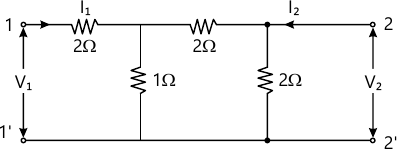7 M
7 (b) Define Y-parameters and draw its equivalent circuit.
6 M

8 (a) Determine the voltage transfer function $$\dfrac {v_2}{v_1}$$ for the network shown below.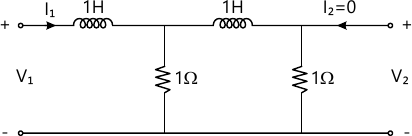7 M
8 (b) Find out the driving point impedance function and voltage ratio transfer function for the given two port N/W.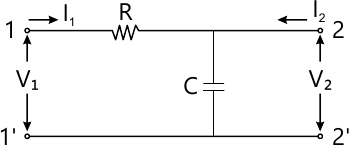6 M

More question papers from Network Theory